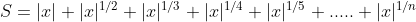# Problem 45761. Sum of terms in a series 1 (★★★)

Given x and n, compute the following sum:where |x| indicates the absolute value of x. Thus, if x = -5 and n = 3, then the sum is (up to n = 3 terms)
|-5|+|-5|^(1/2)+|-5|^(1/3)
= 5+5^(1/2)+5^(1/3)
= 8.946
Avoid using for/while loops.

### Solution Stats

39.26% Correct | 60.74% Incorrect
Last Solution submitted on Aug 03, 2023

### Community Treasure Hunt

Find the treasures in MATLAB Central and discover how the community can help you!

Start Hunting!